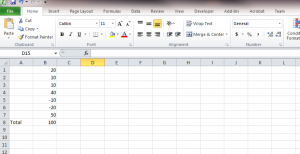VBA To Prevent You From Changing List Total.

There are times while working with an excel list that you would like to delete items of a list without changing the total Sum of that list. Example of this situation often occur when you are reconciling an account and you have various positive and negative values that offset each other.  In this small article I will illustrate how you can use Excel and VBA to warn you when your cell deletion has cause the total value of a list to change. Lets take a quick look on how you would tackle this.

Imagine you had  the following list in a excel sheet.You can tell by looking at this list that you can easily delete the positive 20 and the -20  without changing the total sum of the list. In addition, we can also remove the -10 and 10.  However, what if the list was a list of 100 rows and you would like to continuously remove items without changing the totals. Well that’s where VBA would come in. The total of this list is 100 as you can tell by looking at cell B8. I will go into the developer tab in Excel and click on Visual Basic. I  will then find “Sheet1” in the VBA project window usually on the left hand side. Double click on Sheet1(Sheet1) to open the editor on the right hand side. From the drop down menus on the top right hand side , select “Worksheet” and “SelectionChange” respectively.  Finally, add code to the “SelectionChange” event of Sheet1 so that it fires this event every time we change something on this sheet.

```private Sub Worksheet_SelectionChange(ByVal Target As Range)

Dim CellValue As String

CellValue = Range("B8").Value
If CellValue <> "100" Then
MsgBox ("OOPS GO BACK")

End If
End Sub
```

We can quickly step through the code, there is not much to it. First we  cast  the CellValue  variable as a string. Than we are assigning the value from cell B8 to the variable CellValue. Finally we are testing the CellValue variable to see if the amount is not equal to the amount we don’t want to change, in this case 100. Thats all there is to it.

Pretty simple, but helpful,  leave me some comments below. I would love to hear what yout thoughts are on this post.